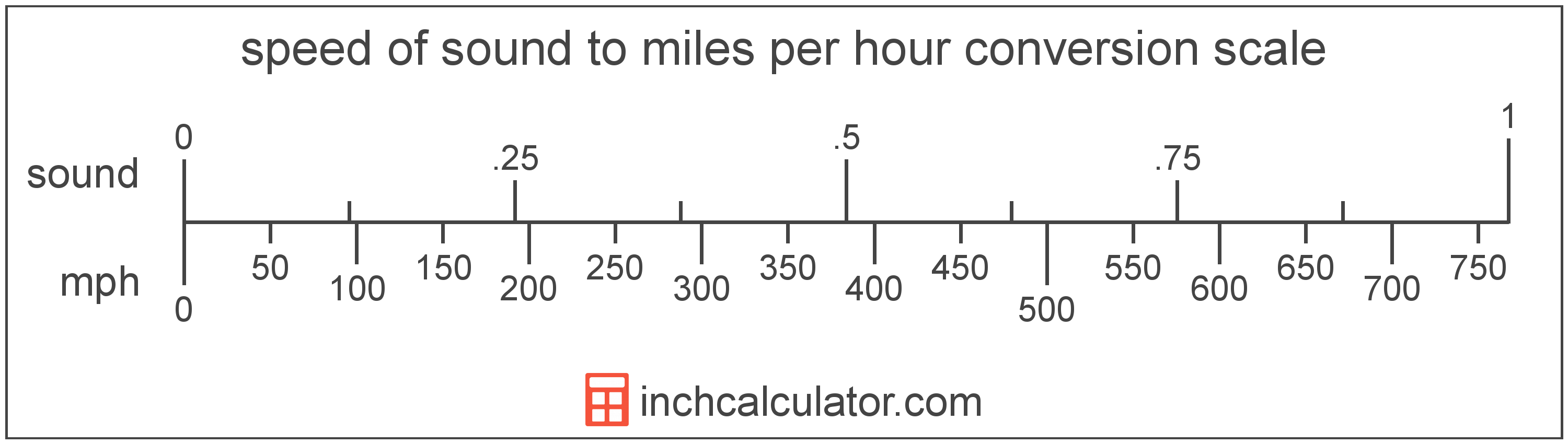# Speed of Sound to Miles per Hour Converter

Enter the speed in speed of sound below to get the value converted to miles per hour.

Results in Miles per Hour:1 sound = 767.269148 mph

Do you want to convert miles per hour to speed of sound?

## How to Convert Speed of Sound to Miles per Hour

To convert a measurement in speed of sound to a measurement in miles per hour, multiply the speed by the following conversion ratio: 767.269148 miles per hour/speed of sound.

Since one speed of sound is equal to 767.269148 miles per hour, you can use this simple formula to convert:

miles per hour = speed of sound × 767.269148

The speed in miles per hour is equal to the speed in speed of sound multiplied by 767.269148.

For example, here's how to convert 5 speed of sound to miles per hour using the formula above.
miles per hour = (5 sound × 767.269148) = 3,836.345741 mphSpeed of sound and miles per hour are both units used to measure speed. Keep reading to learn more about each unit of measure.

## What Is the Speed of Sound?

The speed of sound is the distance a sound wave travels through an elastic medium. The speed of sound through air at 20 °C is equal to 343 meters per second, or roughly 767 miles per hour.

Speed of sound can be abbreviated as sound; for example, 1 speed of sound can be written as 1 sound.

## What Are Miles per Hour?

Miles per hour are a measurement of speed expressing the distance traveled in miles in one hour.

The mile per hour is a US customary and imperial unit of speed. Miles per hour can be abbreviated as mph, and are also sometimes abbreviated as mi/h or MPH. For example, 1 mile per hour can be written as 1 mph, 1 mi/h, or 1 MPH.

Miles per hour can be expressed using the formula:
vmph = dmi / thr

The velocity in miles per hour is equal to the distance in miles divided by time in hours.

## Speed of Sound to Mile per Hour Conversion Table

Table showing various speed of sound measurements converted to miles per hour.
Speed Of Sound Miles Per Hour
1 sound 767.27 mph
2 sound 1,535 mph
3 sound 2,302 mph
4 sound 3,069 mph
5 sound 3,836 mph
6 sound 4,604 mph
7 sound 5,371 mph
8 sound 6,138 mph
9 sound 6,905 mph
10 sound 7,673 mph
11 sound 8,440 mph
12 sound 9,207 mph
13 sound 9,974 mph
14 sound 10,742 mph
15 sound 11,509 mph
16 sound 12,276 mph
17 sound 13,044 mph
18 sound 13,811 mph
19 sound 14,578 mph
20 sound 15,345 mph
21 sound 16,113 mph
22 sound 16,880 mph
23 sound 17,647 mph
24 sound 18,414 mph
25 sound 19,182 mph
26 sound 19,949 mph
27 sound 20,716 mph
28 sound 21,484 mph
29 sound 22,251 mph
30 sound 23,018 mph
31 sound 23,785 mph
32 sound 24,553 mph
33 sound 25,320 mph
34 sound 26,087 mph
35 sound 26,854 mph
36 sound 27,622 mph
37 sound 28,389 mph
38 sound 29,156 mph
39 sound 29,923 mph
40 sound 30,691 mph

## References

1. CK-12, Speed of Sound, https://www.ck12.org/physics/speed-of-sound/lesson/Speed-of-Sound-MS-PS/
2. Wikipedia, Miles per hour, https://en.wikipedia.org/wiki/Miles_per_hour